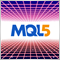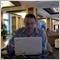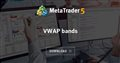# Indicators with alerts/signal - page 157810

Sergey Golubev:

I removed decompiled code from your attachment.
For information:

----------------------

Freelance, decompilation, the rules, examples of the decompiled code:
https://www.mql5.com/en/forum/203200

----------------------

Similar indicator on the forum (as ex4 files only sorry):

Sorry about that, here is the .ex4 version. Could someone tell me how I could write an indicator which will use the iCustom function to read the Trend Direction and Force Index indicator's value and play a sound if it reaches 1 or -1?Moderator
13504

Martin_Gale:
Sorry about that, here is the .ex4 version. Could someone tell me how I could write an indicator which will use the iCustom function to read the Trend Direction and Force Index indicator's value and play a sound if it reaches 1 or -1?

Please post the original MQ4 file.8

Am new here. Am trying to setup my own Expert Advisor/TradingRobot. Any help?Moderator
125813

F1DFD38F:
Am new here. Am trying to setup my own Expert Advisor/TradingRobot. Any help?10

Marco vd Heijden:

Please post the original MQ4 file.

Here is the file, it would be great if we could add a sound alert any time the indicator value reaches -1 or 1.

<decompiled code removed>37

`Hi, does anyone have the Market_Statistics indicator for Metatrader 5? The indicator of which I speak I found it on Metatrader 4, it is Vwap and relative standard deviations. Can you help me ?`379622

birbante16:VWAP bands
• www.mql5.com
This indicator is similar to Bollinger bands, but it uses volume weighted average as the central value of the bands.37

`Thanks William but that's not what I indicated, it's similar but not the same. It does not use Bollinger bands but standard deviations. I have the indicator on my MT4 but I wanted the same on the MT5. If I provide the code, can someone translate it for the Metatrader 5?`37

```//+------------------------------------------------------------------+
//|                                       Market_Statistics_v4_2.mq4 |
//|                                             akifusenet@gmail.com |
//| Volume histogram implementation is based on Vadim Shumilov       |
//| (DrShumiloff)'s VolumesHist2.3 indicator. Other concepts are     |
//|                                                                  |
//| History:                                                         |
//| 17.01.2010: v4.2=>Small Bugfix [zero division possibility ]      |
//|                 =>When time period change call deinit and init   |
//| 09.09.2009: v4.1=>Small bugfix [delete startDate correct prefix] |
//| 09.09.2009: v4=>Instead of fixed start date now we have a        |
//|               =>relative startdate like 2 days back at 22:00     |
//| 02.09.2009: v3=>Calculation done only at the start of a new bar  |
//|               =>implementation is corrected                      |
//|               =>histogram defaulted 50 bar sd3show made true     |
//| 10.08.2009: v2=>End date added.SD2Show defaulted to true         |
//|               =>Show/disable options added for every line        |
//| 06.08.2009: v1=>Initial release                                  |
//|                                                                  |
//+------------------------------------------------------------------+

#property indicator_chart_window

#property indicator_buffers 8

#property indicator_color1 Orange      //PVP
#property indicator_width1 2

#property indicator_color2 Aqua        //VWAP
#property indicator_width2 2

#property indicator_color3 Yellow       //SD1Pos
#property indicator_width3 1
#property indicator_style3 2

#property indicator_color4 White         //SD1Neg
#property indicator_width4 1
#property indicator_style4 2

#property indicator_color5 Yellow//SD2Pos
#property indicator_width5 1
#property indicator_style5 2

#property indicator_color6 White     //SD2Neg
#property indicator_width6 1
#property indicator_style6 2

#property indicator_color7 Yellow   //SD2Pos
#property indicator_width7 1
#property indicator_style7 2

#property indicator_color8 White   //SD2Neg
#property indicator_width8 1
#property indicator_style8 2

//---- input parameters
extern string  label="Market_Statistics_1";
extern int     daysBack=1;
extern int     startHour=22;
extern int     startMinute=00;
extern int     HistogramAmplitude = 50;
extern bool    Show_SD1 = true;
extern bool    Show_SD2 = true;
extern bool    Show_SD3 = true;
extern bool    Show_Histogram = true;
extern bool    Show_PVP = true;
extern bool    Show_VWAP = true;

//---- buffers
double PVP[];
double VWAP[];
double SD1Pos[];
double SD1Neg[];
double SD2Pos[];
double SD2Neg[];
double SD3Pos[];
double SD3Neg[];

double Hist[]; // drawn specifically

int      period         =-2;
double   OpenTime       = 0;  // To check if we have a new bar
int      Bars_Back      = 0;  // Shows the starting bar for given date
int      items;               // numbers of items inside volume histogram
double   SD;                  // standart deviation
string   OBJECT_PREFIX;

// Finds the bar number for the given date
int FindStartIndex()
{
int dayofweektoday= TimeDayOfWeek(Time);
int days=0;

for (int i=1; i<=Bars; i++) {

if ((TimeDayOfWeek(Time[i])!=dayofweektoday)||(daysBack==0)) {
days++;
dayofweektoday=TimeDayOfWeek(Time[i]);

if ((daysBack==days)||(daysBack==0))
{
while ((TimeHour(Time[i])>startHour)||(TimeMinute(Time[i])>startMinute))
{
i++;
}

return (i);
}
}
}

return( 0);
}

int init()
{
OBJECT_PREFIX = label+"_VolumeHistogram_" + DoubleToStr(Time[FindStartIndex()],0)+"_" ;

//---- indicators
IndicatorBuffers(8);

if (Show_PVP==true) SetIndexStyle(0,DRAW_LINE);
else  SetIndexStyle(0,DRAW_NONE);
SetIndexLabel(0,"PVP");
SetIndexBuffer(0,PVP);

if (Show_VWAP==true) SetIndexStyle(1,DRAW_LINE);
else  SetIndexStyle(1,DRAW_NONE);
SetIndexLabel(1,"VWAP");
SetIndexBuffer(1,VWAP);

if (Show_SD1==true) SetIndexStyle(2,DRAW_LINE);
else  SetIndexStyle(2,DRAW_NONE);
SetIndexLabel(2,"SD1Pos");
SetIndexBuffer(2,SD1Pos);

if (Show_SD1==true) SetIndexStyle(3,DRAW_LINE);
else  SetIndexStyle(3,DRAW_NONE);
SetIndexLabel(3,"SD1Neg");
SetIndexBuffer(3,SD1Neg);

if (Show_SD2==true) SetIndexStyle(4,DRAW_LINE);
else  SetIndexStyle(4,DRAW_NONE);
SetIndexLabel(4,"SD2Pos");
SetIndexBuffer(4,SD2Pos);

if (Show_SD2==true) SetIndexStyle(5,DRAW_LINE);
else  SetIndexStyle(5,DRAW_NONE);
SetIndexLabel(5,"SD2Neg");
SetIndexBuffer(5,SD2Neg);

if (Show_SD3==true) SetIndexStyle(6,DRAW_LINE);
else  SetIndexStyle(6,DRAW_NONE);
SetIndexLabel(6,"SD3Pos");
SetIndexBuffer(6,SD3Pos);

if (Show_SD3==true) SetIndexStyle(7,DRAW_LINE);
else  SetIndexStyle(7,DRAW_NONE);
SetIndexLabel(7,"SD3Neg");
SetIndexBuffer(7,SD3Neg);

string short_name="Market_Statistics";
IndicatorShortName(short_name);

return(0);
}

// Delete all objects with given prefix
void ObDeleteObjectsByPrefix(string Prefix)
{
int L = StringLen(Prefix);
int i = 0;
while(i < ObjectsTotal())
{
string ObjName = ObjectName(i);
if(StringSubstr(ObjName, 0, L) != Prefix)
{
i++;
continue;
}
ObjectDelete(ObjName);
}
}

int start()
{
double TotalVolume=0;
double TotalPV=0;
int n;

if (period!=Period()&&(period!=-2))
{
Print("Period has been changed. Recalculation needed");
deinit();
init();
}
period=Period();

if (OpenTime != Time)
{
Bars_Back=FindStartIndex();
if (Bars_Back!=0)
{
ObjectSet(label+"Starting_Time", OBJPROP_TIME1, Time[Bars_Back]);
ObjectSet(label+"Starting_Time", OBJPROP_COLOR, Red);
ObjectCreate(label+"Starting_Time", OBJ_VLINE, 0, Time[Bars_Back], 0);
}

OpenTime = Time;

double max = High[iHighest( NULL , 0, MODE_HIGH, Bars_Back, 0)];
double min =  Low[ iLowest( NULL , 0, MODE_LOW,  Bars_Back, 0)];
items = MathRound((max - min) / Point);

ArrayResize(Hist, items);
ArrayInitialize(Hist, 0);

TotalVolume=0;
TotalPV=0;
for (int i = Bars_Back; i >= 1; i--)
{

double t1 = Low[i], t2 = Open[i], t3 = Close[i], t4 = High[i];
if (t2 > t3) {t3 = Open[i]; t2 = Close[i];}
double totalRange = 2*(t4 - t1) - t3 + t2;

if (totalRange != 0.0)
{
for (double Price_i = t1; Price_i <= t4; Price_i += Point)
{
n = MathRound((Price_i - min) / Point);

if (t1 <= Price_i && Price_i <  t2)
{
Hist[n] += MathRound(Volume[i]*2*(t2-t1)/totalRange);
}
if (t2 <= Price_i && Price_i <= t3)
{
Hist[n] += MathRound(Volume[i]*(t3-t2)/totalRange);
}
if (t3 <  Price_i && Price_i <= t4)
{
Hist[n] += MathRound(Volume[i]*2*(t4-t3)/totalRange);
}
}//for
}else
{
// Check if all values are equal to each other
n = MathRound((t3 - min) / Point);
Hist[n] += Volume[i];
}//if

// use H+L+C/3 as average price
TotalPV+=Volume[i]*((Low[i]+High[i]+Close[i])/3);
TotalVolume+=Volume[i];

if (i==Bars_Back) PVP[i]=Close[i];
else PVP[i]=min+ArrayMaximum(Hist)*Point;

if (i==Bars_Back) VWAP[i]=Close[i];
else
{
if (TotalVolume!=0) VWAP[i]=TotalPV/TotalVolume;
}

SD=0;
for (int k = Bars_Back; k >= i; k--)
{
double avg=(High[k]+Close[k]+Low[k])/3;
double diff=avg-VWAP[i];
if (TotalVolume!=0) SD+=(Volume[k]/TotalVolume)*(diff*diff);
}
SD=MathSqrt(SD);
SD1Pos[i]=VWAP[i]+SD;
SD1Neg[i]=VWAP[i]-SD;
SD2Pos[i]=SD1Pos[i]+SD;
SD2Neg[i]=SD1Neg[i]-SD;
SD3Pos[i]=SD2Pos[i]+SD;
SD3Neg[i]=SD2Neg[i]-SD;

}//for BARS BACK

ObDeleteObjectsByPrefix(OBJECT_PREFIX);
if (Show_Histogram)
{
int MaxVolume = Hist[ArrayMaximum(Hist)];
int multiplier;
for (i = 0; i <= items; i++)
{
// Protection if we have less bar than amplitude yet
if (Bars_Back<HistogramAmplitude) multiplier=Bars_Back;
else multiplier=HistogramAmplitude;

if (MaxVolume != 0) Hist[i] = MathRound(multiplier * Hist[i] / MaxVolume );

if (Hist[i] > 0)
{
int time_i = Bars_Back-Hist[i];
if (time_i>=0)
{
ObjectCreate(OBJECT_PREFIX+i, OBJ_RECTANGLE, 0, Time[Bars_Back], min + i*Point, Time[time_i], min + (i+1)*Point);
ObjectSet(OBJECT_PREFIX+i, OBJPROP_STYLE, DRAW_HISTOGRAM);
ObjectSet(OBJECT_PREFIX+i, OBJPROP_COLOR, Teal);
ObjectSet(OBJECT_PREFIX+i, OBJPROP_BACK, true);
}//if
} //if
}//for

}//if show histogram
}//MAIN IF BAR START

return(0);
}

int deinit()
{
ObDeleteObjectsByPrefix(OBJECT_PREFIX);
ObjectDelete(label+"Starting_Time");
return(0);
}

```

```This is the code on the MT4. Does Anyone know how to translate it for the Metatrader 5? I would be very
grateful```